Journal topic
Biogeosciences, 17, 3875–3890, 2020
https://doi.org/10.5194/bg-17-3875-2020Biogeosciences, 17, 3875–3890, 2020
https://doi.org/10.5194/bg-17-3875-2020Research article 31 Jul 2020

Research article | 31 Jul 2020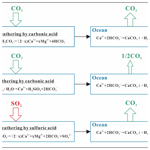# Temporary and net sinks of atmospheric CO2 due to chemical weathering in subtropical catchment with mixing carbonate and silicate lithology

Temporary and net sinks of atmospheric CO2 due to chemical weathering in subtropical catchment with mixing carbonate and silicate lithology
Yingjie Cao1,3,4, Yingxue Xuan1,2, Changyuan Tang1,2,3, Shuai Guan5, and Yisheng Peng1,3 Yingjie Cao et al.
• 1School of Environmental Science and Engineering, Sun Yat-Sen University, Guangzhou, China
• 2School of Geography and Planning, Sun Yat-Sen University, Guangzhou, China
• 3Guangdong Provincial Key Laboratory of Environmental Pollution Control and Remediation Technology, Sun Yat-Sen University, Guangzhou, China
• 4Southern Marine Science and Engineering Guangdong Laboratory, Zhuhai, China
• 5Guangdong Research Institute of Water Resource and Hydropower, Guangzhou, China

Correspondence: Changyuan Tang (changyuan_tang@163.com, tangchy3@mail.sysu.edu.cn)

Abstract

The study provided the major ion chemistry, chemical weathering rates and temporary and net CO2 sinks in the Bei Jiang, which was characterized as a hyperactive region with high chemical weathering rates, carbonate and silicate mixing lithology, and abundant sulfuric acid chemical weathering agent of acid deposition and acid mining drainage (AMD) origins. The total chemical weathering rate of 85.46 t km−2 a−1 was comparable to that of other rivers in the hyperactive zones between the latitudes 0 and 30. A carbonate weathering rate of 61.15 t km−2 a−1 contributed to about 70 % of the total. The lithology, runoff, and geomorphology had a significant influence on the chemical weathering rate. The proportion of carbonate outcrops had a significant positive correlation with the chemical weathering rate. Due to the interaction between dilution and compensation effect, a significant positive linear relationship was detected between runoff and total carbonate and silicate weathering rates. The geomorphology factors such as catchment area, average slope, and hypsometric integral value (HI) had nonlinear correlation with chemical weathering rate and showed significant scale effect, which revealed the complexity in chemical weathering processes. Dissolved inorganic carbon (DIC) apportionment showed that CCW (carbonate weathering by CO2) was the dominant origin of DIC (35 %–87 %). SCW (carbonate weathering by H2SO4) (3 %–15 %) and CSW (silicate weathering by CO2) (7 %–59 %) were non-negligible processes. The temporary CO2 sink was 823.41×103 mol km−2 a−1. Compared with the temporary sink, the net sink of CO2 for the Bei Jiang was approximately 23.18×103 mol km−2 a−1 of CO2 and was about 2.82 % of the “temporary” CO2 sink. Human activities (sulfur acid deposition and AMD) dramatically decreased the CO2 net sink, even making chemical weathering a CO2 source to the atmosphere.

1 Introduction

Chemical weathering driven by weak carbonic acid (H2CO3) that originates from atmosphere CO2 or soil respiration under natural conditions is a fundamental geochemical process regulating the atmosphere–land–ocean carbon fluxes and Earth's climate (Guo et al., 2015). Carbonate and silicate weathering define the two typical categories of chemical weathering. From the view of the global carbon cycle, the CO2 consumption due to carbonate weathering is recognized as the “temporary” sink because the flux of CO2 consumed by carbonate dissolution on the continents is balanced by the flux of CO2 released into the atmosphere from the oceans by carbonate precipitation on the geological timescale (Cao et al., 2015; Garrels, 1983). The consumption of CO2 during chemical weathering of silicate rocks has been regarded as the net sink of CO2 and regulates the global carbon cycle (Hartmann et al., 2009, 2014b; Kempe and Degens, 1985; Lenton and Britton, 2006). Thus in a carbonate–silicate mixing catchment, it is essential to distinguish proportions of the two most important lithological groups, i.e., carbonates and silicates, and evaluate the net CO2 sink due to chemical weathering of silicate (Hartmann et al., 2009).

In addition to the chemical weathering induced by H2CO3, sulfuric acid (H2SO4) of anthropogenic origins produced by sulfide oxidation such as acid deposition caused by fossil fuel burning and acid mining discharge (AMD) also becomes an important chemical weathering agent in the catchment scale. Many studies have shown the importance of sulfide oxidation and subsequent dissolution of other minerals by the resulting sulfuric acid at catchment scale (Hercod et al., 1998; Spence and Telmer, 2005). Depending on the fate of sulfate in the oceans, sulfide oxidation coupled with carbonate dissolution could facilitate a release of CO2 to the atmosphere (Spence and Telmer, 2005). The carbonate weathering by H2SO4 plays a very important role in quantifying and validating the ultimate CO2 consumption rate. Thus, under the influence of human activities, the combination of silicate weathering by H2CO3 and carbonate weathering by H2SO4 controlled the net sink of atmospheric CO2.

Numerous studies on chemical weathering of larger rivers have been carried out to examine hydrochemical characteristics, chemical erosion and CO2 consumption rates, and long-term climatic evolution of the Earth, for example for the Yangtze River (Chen et al., 2002; Ran et al., 2010), the Huang He (Zhang et al., 1995), the Pearl River (Gao et al., 2009; Xu and Liu, 2010; Zhang et al., 2007), the Huai River (Zhang et al., 2011), the rivers of the Qinghai–Tibetan Plateau (Jiang et al., 2018; Li et al., 2011; Wu et al., 2008), the Mekong River (Li et al., 2014), the rivers of the Alpine region (Donnini et al., 2016), the Sorocaba River (Fernandes et al., 2016), the rivers of Baltic Sea catchment (Sun et al., 2017), the Amazon River (Gibbs, 1972; Mortatti and Probst, 2003; Stallard and Edmond, 1981; Stallard and Edmond, 1983, 1987), the Lena River (Huh and Edmond, 1999), and the Orinoco River (Mora et al., 2010). For simplicity of calculation, most of the research has ignored sulfuric-acid-induced chemical weathering and resulted in an overestimation of CO2 sink. To overcome this shortcoming of the traditional mass balance method, we applied a dissolved inorganic carbon (DIC) source apportionment procedure to discriminate the contribution of sulfuric-acid-induced chemical weathering to validate the temporary and net sink of CO2 in a typical hyperactive region with carbonate–silicate mixing lithology to give a further understanding of basin-scale chemical weathering estimation.

About half of the global CO2 sequestration due to chemical weathering occurs in warm and high-runoff regions (Ludwig et al., 1998), called the hyperactive regions and hot spots (Meybeck et al., 2006). The Pearl River located in the subtropical area in south China includes three principal rivers: the Xi Jiang, Bei Jiang, and Dong Jiang. The warm and wet climatic conditions make the Pearl River a hyperactive region in China. The three river basins have distinct geological conditions. The Xi Jiang is characterized as the carbonate-dominated area, and the Dong Jiang has silicate as the main rock type. The Bei Jiang, which is the second largest tributary of the Pearl River, is characterized as a typical carbonate–silicate mixing basin. In addition, as the severe acid deposition (Larssen et al., 2006) and active mining area (Li et al., 2019), chemical weathering induced by sulfuric acid make it necessary to reevaluate the temporary and net sinks of atmospheric CO2. Thus, the Bei Jiang in southeast China with a typical subtropical monsoon climate and carbonate–silicate mixing geologic settings was selected as the study area. The three main objectives are summarized as follows: (1) reveal spatial–temporal variations in major element chemistry of the river water, (2) calculate the chemical weathering rate and unravel the controlling factors on chemical weathering processes, and (3) determine the temporary sink of CO2 and evaluate the influence of sulfide oxidation on the net sink of CO2 by DIC apportionment.

2 Study area

The Bei Jiang basin, which is the second largest tributary of the Pearl River basin, is located in the southeast of China (Fig. 1). It covers an area of 52 068 km2 and has a total length of 573 km. The river basin is located in subtropical monsoon climate zone, with the mean annual temperature across the drainage basin ranging from 14 to 22 C and the mean annual precipitation ranging from 1390 to 2475 mm. The average annual runoff is 51×109 m3, with 70 %–80 % of the flux occurring from April to September. This can be attributed to the fact that more than 70 % of the annual precipitation (about 1800 mm yr−1) is concentrated in the wet season (April to September).

Lithology in the river basin is composed of limestone, sandstone, gneiss, and glutenite. In the upper basin, carbonate rock (mainly of limestone) outcrops in the west and center, while sandstone of the Devonian era and mudstone of the Paleogene era outcrop in the east of the upper stream. In the middle of basin, limestone and sandstone cover most of the area, and Cretaceous volcanic rocks are found in the tributary (Lian Jiang), mainly granite. In the lower basin, Archean metamorphic rocks outcrop in the west and are composed of gneiss and schist; sandstone covers the rest of area of the lower basin. Quaternary sediments are scattered along the main stream of the river. The carbonate and silicate rock outcrops in the Bei Jiang basin are 10 737 km2 (28 %) and 24 687 km2 (65 %), respectively.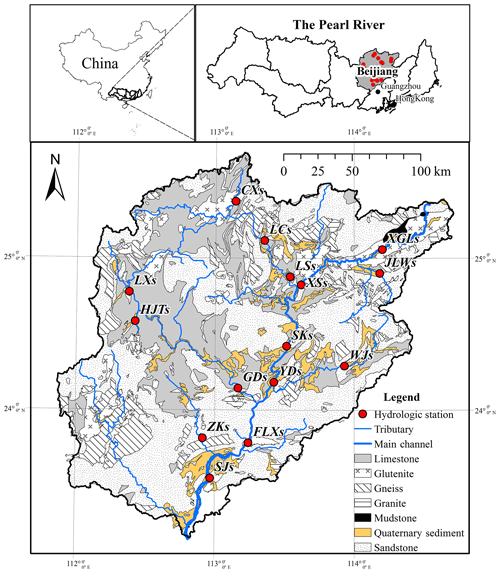Figure 1Geology map and sampling point in the Bei Jiang basin (produced by ArcGIS).

3 Materials and methods

## 3.1 Sampling procedure and laboratory analysis

Water samples were collected monthly at 15 hydrologic stations from January to December in 2015 (Fig. 1). The river waters were sampled by a portable organic class water sampler along the middle thread of the channel on the first day of each month. In addition, to discriminate the contribution of rain inputs, the daily rainwater was also sampled at five stations (SJs, FLXs, YDs, XSs, and XGLs) along the main stream. The rainwater collector is consisted of a funnel with a diameter of 20 cm and a 5 L plastic bottle. A rubber ball is set up in the funnel to prevent evaporation. All the river and rainwater were filtered through a 0.45 µm glass fiber filter and stored in 100 mL tubes and stored below 4 C until analysis.

Electric conductivity (EC), pH, and temperature (T) were measured using a multi-parameter water quality meter (HACH-HQ40Q), and alkalinity (${\mathrm{HCO}}_{\mathrm{3}}^{-}$) was measured in filtered water samples by titration in situ. The dissolved SiO2 was measured with the molybdenum yellow method and was analyzed using an ultraviolet spectrophotometer (Shimadzu UV-2600). The cations (Na+, K+, Ca2+, Mg2+) and anions (Cl, ${\mathrm{SO}}_{\mathrm{4}}^{\mathrm{2}-}$) were analyzed with ion chromatography (Thermo Fisher ICS-900) with a limit of detection (L.O.D) of 0.01 mg L−1. Reference, blank, and replicate samples were employed to check the accuracy of all the analyses, and the relative standard deviations of all the analyses were within ±5 %. The electrical balance (E.B.) defined by the equation $\mathrm{E}.\mathrm{B}.=\frac{\mathrm{meq}\left(\mathrm{sum}\phantom{\rule{0.25em}{0ex}}\mathrm{of}\phantom{\rule{0.25em}{0ex}}\mathrm{cations}\right)-\mathrm{meq}\left(\mathrm{sum}\phantom{\rule{0.25em}{0ex}}\mathrm{of}\phantom{\rule{0.25em}{0ex}}\mathrm{anions}\right)}{\mathrm{meq}\left(\mathrm{sum}\phantom{\rule{0.25em}{0ex}}\mathrm{of}\phantom{\rule{0.25em}{0ex}}\mathrm{cations}\phantom{\rule{0.25em}{0ex}}\mathrm{and}\phantom{\rule{0.25em}{0ex}}\mathrm{anions}\right)}×\mathrm{100}$ of the water samples was less than 5 %.

## 3.2 Calculation procedure

### 3.2.1 Chemical weathering rates

The mass balance equation for element X in the dissolved load can be expressed as (Galy and France-Lanord, 1999)

$\begin{array}{}\text{(1)}& \left[X{\right]}_{\mathrm{riv}}=\left[X{\right]}_{\mathrm{pre}}+\left[X{\right]}_{\mathrm{eva}}+\left[X{\right]}_{\mathrm{sil}}+\left[X{\right]}_{\mathrm{car}}+\left[X{\right]}_{\mathrm{anth}}.\end{array}$

Here [X] denotes the elements of Ca2+, Mg2+, Na+, K+, Cl, ${\mathrm{SO}}_{\mathrm{4}}^{\mathrm{2}-}$, and ${\mathrm{HCO}}_{\mathrm{3}}^{-}$ in millimoles per liter. The subscripts riv, pre, eva, sil, car, and anth denote the river, precipitation source, evaporite source, silicate source, carbonate source, and anthropogenic source.

In the study area, the anthropogenic source of major ions except for ${\mathrm{SO}}_{\mathrm{4}}^{\mathrm{2}-}$ was ignored due to the following two reasons. (1) Two main characteristics of much-polluted rivers are that total dissolved solid (TDS) is greater than 500 mg L−1 and the ${\mathrm{Cl}}^{-}/{\mathrm{Na}}^{+}$ molar ratio is greater than that of sea salts (about 1.16) (Cao et al., 2016a; Gaillardet et al., 1999). The TDS in the study area ranged from 73.79 to 230.16 mg L−1, and the low TDS implied that the anthropogenic origins of major ions could be ignored in the study. However, the Bei Jiang is characterized as a typical region suffering from severe acid deposition (Larssen et al., 2006) and are active mining area (Li et al., 2019). The acid deposition and acid mining discharge contribute to the highest concentration of ${\mathrm{SO}}_{\mathrm{4}}^{\mathrm{2}-}$. (2) Natural origin of ${\mathrm{SO}}_{\mathrm{4}}^{\mathrm{2}-}$ is the dissolution of evaporite, such as gypsum, while no evaporite was found in the study area. If ${\mathrm{SO}}_{\mathrm{4}}^{\mathrm{2}-}$ comes from the gypsum dissolution, the ratios of Ca2+ and ${\mathrm{SO}}_{\mathrm{4}}^{\mathrm{2}-}$ should be close to 1 : 1. The stoichiometric analysis (Fig. 2) showed that the ratio of Ca2+ and ${\mathrm{SO}}_{\mathrm{4}}^{\mathrm{2}-}$ deviated from 1 : 1 and also proved this point.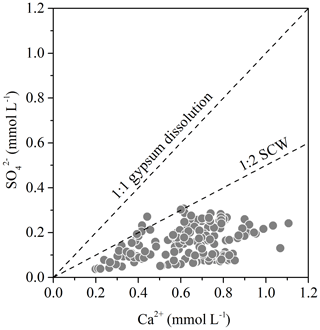Figure 2Stoichiometric relationship between Ca2+ and ${\mathrm{SO}}_{\mathrm{4}}^{\mathrm{2}-}$. The “SCW” means carbonate weathering induced by sulfuric acid.

Therefore, on the basis of the theory of rock chemical weathering and ignoring the anthropogenic origins of major ions (except for ${\mathrm{SO}}_{\mathrm{4}}^{\mathrm{2}-}$), the major elements of river water can be simplified as follows.

$\begin{array}{}\text{(2)}& \left[{\mathrm{Cl}}^{-}{\right]}_{\mathrm{riv}}=\left[{\mathrm{Cl}}^{-}{\right]}_{\mathrm{pre}}+\left[{\mathrm{Cl}}^{-}{\right]}_{\mathrm{eva}}\text{(3)}& \left[{\mathrm{K}}^{+}{\right]}_{\mathrm{riv}}=\left[{\mathrm{K}}^{+}{\right]}_{\mathrm{pre}}+\left[{\mathrm{K}}^{+}{\right]}_{\mathrm{sil}}\text{(4)}& \left[{\mathrm{Na}}^{+}{\right]}_{\mathrm{riv}}=\left[{\mathrm{Na}}^{+}{\right]}_{\mathrm{pre}}+\left[{\mathrm{Na}}^{+}{\right]}_{\mathrm{eva}}+\left[{\mathrm{Na}}^{+}{\right]}_{\mathrm{sil}}\text{(5)}& \left[{\mathrm{Ca}}^{\mathrm{2}+}{\right]}_{\mathrm{riv}}=\left[{\mathrm{Ca}}^{\mathrm{2}+}{\right]}_{\mathrm{pre}}+\left[{\mathrm{Ca}}^{\mathrm{2}+}{\right]}_{\mathrm{sil}}+\left[{\mathrm{Ca}}^{\mathrm{2}+}{\right]}_{\mathrm{car}}\text{(6)}& \left[{\mathrm{Mg}}^{\mathrm{2}+}{\right]}_{\mathrm{riv}}=\left[{\mathrm{Mg}}^{\mathrm{2}+}{\right]}_{\mathrm{pre}}+\left[{\mathrm{Mg}}^{\mathrm{2}+}{\right]}_{\mathrm{sil}}+\left[{\mathrm{Mg}}^{\mathrm{2}+}{\right]}_{\mathrm{car}}\text{(7)}& \begin{array}{rl}\left[{\mathrm{HCO}}_{\mathrm{3}}^{-}{\right]}_{\mathrm{sil}}& =\left[{\mathrm{K}}^{+}{\right]}_{\mathrm{sil}}+\left[{\mathrm{Na}}^{+}{\right]}_{\mathrm{sil}}+\mathrm{2}\left[{\mathrm{Mg}}^{\mathrm{2}+}{\right]}_{\mathrm{sil}}\\ & +\mathrm{2}\left[{\mathrm{Ca}}^{\mathrm{2}+}{\right]}_{\mathrm{sil}}\end{array}\text{(8)}& \left[{\mathrm{HCO}}_{\mathrm{3}}^{-}{\right]}_{\mathrm{car}}=\left[{\mathrm{HCO}}_{\mathrm{3}}^{-}{\right]}_{\mathrm{riv}}-\left[{\mathrm{HCO}}_{\mathrm{3}}^{-}{\right]}_{\mathrm{sil}}\text{(9)}& \left[{\mathrm{SO}}_{\mathrm{4}}^{\mathrm{2}-}{\right]}_{\mathrm{riv}}=\left[{\mathrm{SO}}_{\mathrm{4}}^{\mathrm{2}-}{\right]}_{\mathrm{pre}}+\left[{\mathrm{SO}}_{\mathrm{4}}^{\mathrm{2}-}{\right]}_{\mathrm{anth}}\end{array}$

Firstly, the measured ion concentrations of the rainwater are rectified by evaporation coefficient $\mathit{\alpha }=\mathrm{0.63}=P/R$ (with P the precipitation and R the runoff), and the contributions of atmospheric precipitation are calculated. Secondly, the molar ratios of ${\mathrm{Ca}}^{\mathrm{2}+}/{\mathrm{Na}}^{+}$ (0.4) and ${\mathrm{Mg}}^{\mathrm{2}+}/{\mathrm{Na}}^{+}$ (0.2) for the silicate end-member (Zhang et al., 2007) are used to calculate the contribution of Ca2+ and Mg2+ from silicate weathering, and then, residual Ca2+ and Mg2+ were attributed to carbonate weathering. For monthly data, the contributions of different sources can be calculated as follows.

$\begin{array}{}\text{(10)}& {R}_{\mathrm{car}}=\left(\left[{\mathrm{Ca}}^{\mathrm{2}+}{\right]}_{\mathrm{car}}+\left[{\mathrm{Mg}}^{\mathrm{2}+}{\right]}_{\mathrm{car}}\right)/S×\mathrm{100}\phantom{\rule{0.125em}{0ex}}\mathit{%}\text{(11)}& \begin{array}{rl}{R}_{\mathrm{sil}}& =\left(\left[{\mathrm{K}}^{+}{\right]}_{\mathrm{sil}}+\left[{\mathrm{Na}}^{+}{\right]}_{\mathrm{sil}}+\left[{\mathrm{Ca}}^{\mathrm{2}+}{\right]}_{\mathrm{sil}}\\ & +\left[{\mathrm{Mg}}^{\mathrm{2}+}{\right]}_{\mathrm{sil}}\right)/S×\mathrm{100}\phantom{\rule{0.125em}{0ex}}\mathit{%}\end{array}\text{(12)}& {R}_{\mathrm{eva}}=\left[{\mathrm{Na}}^{+}{\right]}_{\mathrm{eva}}/S×\mathrm{100}\phantom{\rule{0.125em}{0ex}}\mathit{%}\text{(13)}& \begin{array}{rl}{R}_{\mathrm{pre}}& =\left(\left[{\mathrm{K}}^{+}{\right]}_{\mathrm{pre}}+\left[{\mathrm{Na}}^{+}{\right]}_{\mathrm{pre}}+\left[{\mathrm{Ca}}^{\mathrm{2}+}{\right]}_{\mathrm{pre}}\\ & +\left[{\mathrm{Mg}}^{\mathrm{2}+}{\right]}_{\mathrm{pre}}\right)/S×\mathrm{100}\phantom{\rule{0.125em}{0ex}}\mathit{%}\end{array}\text{(14)}& \begin{array}{rl}S& =\left[{\mathrm{Ca}}^{\mathrm{2}+}{\right]}_{\mathrm{car}}+\left[{\mathrm{Mg}}^{\mathrm{2}+}{\right]}_{\mathrm{car}}+\left[{\mathrm{Ca}}^{\mathrm{2}+}{\right]}_{\mathrm{sil}}+\left[{\mathrm{Mg}}^{\mathrm{2}+}{\right]}_{\mathrm{sil}}\\ & +\left[{\mathrm{Na}}^{+}{\right]}_{\mathrm{sil}}+\left[{\mathrm{K}}^{+}{\right]}_{\mathrm{sil}}+\left[{\mathrm{Na}}^{+}{\right]}_{\mathrm{eva}}+\left[{\mathrm{Ca}}^{\mathrm{2}+}{\right]}_{\mathrm{pre}}\\ & +\left[{\mathrm{Mg}}^{\mathrm{2}+}{\right]}_{\mathrm{pre}}+\left[{\mathrm{Na}}^{+}{\right]}_{\mathrm{pre}}+\left[{\mathrm{K}}^{+}{\right]}_{\mathrm{pre}}\end{array}\end{array}$

Here R denotes the proportions of dissolved cations from different sources. S denotes the total concentrations of cations for river water in millimoles per liter.

The total, carbonate and silicate chemical weathering rates (TWR, CWR, and SWR) of a year can be estimated as follows.

$\begin{array}{}\text{(15)}& \begin{array}{rl}\mathrm{CWR}& =\sum _{i=\mathrm{1}}^{n=\mathrm{12}}\left[\left(\mathrm{24}×\left[{\mathrm{Mg}}^{\mathrm{2}+}{\right]}_{\mathrm{car}}+\mathrm{40}×\left[{\mathrm{Ca}}^{\mathrm{2}+}{\right]}_{\mathrm{car}}\\ & +\mathrm{61}×\left[{\mathrm{HCO}}_{\mathrm{3}}^{-}{\right]}_{\mathrm{car}}×\mathrm{0.5}{\right)}_{i}×{Q}_{i}/\left({\mathrm{10}}^{\mathrm{6}}A\right)\right]\end{array}\text{(16)}& \begin{array}{rl}\mathrm{SWR}& =\sum _{i=\mathrm{1}}^{n=\mathrm{12}}\left[\left(\mathrm{39}×\left[{\mathrm{K}}^{+}{\right]}_{\mathrm{sil}}+\mathrm{23}×\left[{\mathrm{Na}}^{+}{\right]}_{\mathrm{sil}}\\ & +\mathrm{24}×\left[{\mathrm{Mg}}^{\mathrm{2}+}{\right]}_{\mathrm{sil}}+\mathrm{40}×\left[{\mathrm{Ca}}^{\mathrm{2}+}{\right]}_{\mathrm{sil}}\\ & +\mathrm{96}×\left[{\mathrm{SiO}}_{\mathrm{2}}{\right]}_{\mathrm{sil}}{\right)}_{i}×{Q}_{i}/\left({\mathrm{10}}^{\mathrm{6}}A\right)\right]\end{array}\text{(17)}& \mathrm{TWR}=\mathrm{CWR}+\mathrm{SWR}\end{array}$

Here TWR, CWR, and SWR in metric tons per square kilometer per year, Qi denotes discharge in cubic meters per month, and A denotes the catchment area in square kilometers.

### 3.2.2 DIC apportionments

In the Bei Jiang, the pH values of water samples ranged from 7.5 to 8.5 with an average of 8.05. Under these pH conditions, the major species of DIC is ${\mathrm{HCO}}_{\mathrm{3}}^{-}$. In addition, ${\mathrm{HCO}}_{\mathrm{3}}^{-}$ accounted for more than 95 % at all sampling sites based on calculation; thus the concentration of ${\mathrm{HCO}}_{\mathrm{3}}^{-}$ (mmol L−1) was used to represent the DIC concentration in this study. The riverine DIC originates from several sources including carbonate minerals, respired soil CO2, and atmospheric CO2, and it could be affected by processes occurring along the water pathways (Khadka et al., 2014; Li et al., 2008). Four dominant weathering processes, including (1) carbonate weathering by carbonic acid (CCW), (2) carbonate weathering by sulfuric acid (SCW), (3) silicate weathering by carbonic acid (CSW), (4) and silicate weathering by sulfuric acid (SSW), can be described by the following reaction equations.

$\begin{array}{}\text{(R1)}& \begin{array}{rl}\mathrm{CCW}:& \left({\mathrm{Ca}}_{\mathrm{2}-X}{\mathrm{Mg}}_{x}\right){\left({\mathrm{CO}}_{\mathrm{3}}\right)}_{\mathrm{2}}+\mathrm{2}{\mathrm{H}}_{\mathrm{2}}{\mathrm{CO}}_{\mathrm{3}}\\ & \to \left(\mathrm{2}-x\right){\mathrm{Ca}}^{\mathrm{2}+}+x{\mathrm{Mg}}^{\mathrm{2}+}+\mathrm{4}{\mathrm{HCO}}_{\mathrm{3}}^{-}\end{array}\text{(R2)}& \begin{array}{rl}\mathrm{SCW}:& \left({\mathrm{Ca}}_{\mathrm{2}-X}{\mathrm{Mg}}_{x}\right){\left({\mathrm{CO}}_{\mathrm{3}}\right)}_{\mathrm{2}}+{\mathrm{H}}_{\mathrm{2}}{\mathrm{SO}}_{\mathrm{4}}\\ & \to \left(\mathrm{2}-x\right){\mathrm{Ca}}^{\mathrm{2}+}+x{\mathrm{Mg}}^{\mathrm{2}+}+\mathrm{2}{\mathrm{HCO}}_{\mathrm{3}}^{-}+{\mathrm{SO}}_{\mathrm{4}}^{\mathrm{2}-}\end{array}\text{(R3)}& \begin{array}{rl}\mathrm{CSW}:& {\mathrm{CaSiO}}_{\mathrm{3}}+\mathrm{2}{\mathrm{H}}_{\mathrm{2}}{\mathrm{CO}}_{\mathrm{3}}+{\mathrm{H}}_{\mathrm{2}}\mathrm{O}\\ & \to {\mathrm{Ca}}^{\mathrm{2}+}+{\mathrm{H}}_{\mathrm{4}}{\mathrm{SiO}}_{\mathrm{4}}+\mathrm{2}{\mathrm{HCO}}_{\mathrm{3}}^{-}\end{array}\text{(R4)}& \begin{array}{rl}\mathrm{SSW}:& {\mathrm{CaSiO}}_{\mathrm{3}}+{\mathrm{H}}_{\mathrm{2}}{\mathrm{SO}}_{\mathrm{4}}+{\mathrm{H}}_{\mathrm{2}}\mathrm{O}\\ & \to {\mathrm{Ca}}^{\mathrm{2}+}+{\mathrm{H}}_{\mathrm{4}}{\mathrm{SiO}}_{\mathrm{4}}+{\mathrm{SO}}_{\mathrm{4}}^{\mathrm{2}-}\end{array}\end{array}$

Here CaSiO3 represents an arbitrary silicate.

According to the study of Galy and France-Lanord (1999) and Spence and Telmer (2005), carbonate and silicate weathering by carbonic acid have the same ratio as carbonate and silicate weathering by sulfuric acid, and for monthly data the mass balance equations are as follows:

$\begin{array}{}\text{(18)}& \left[{\mathrm{SO}}_{\mathrm{4}}^{\mathrm{2}-}{\right]}_{\mathrm{riv}}-\left[{\mathrm{SO}}_{\mathrm{4}}^{\mathrm{2}-}{\right]}_{\mathrm{pre}}=\left[{\mathrm{SO}}_{\mathrm{4}}^{\mathrm{2}-}{\right]}_{\mathrm{SCW}}+\left[{\mathrm{SO}}_{\mathrm{4}}^{\mathrm{2}-}{\right]}_{\mathrm{SSW}},\text{(19)}& \begin{array}{rl}\left[{\mathrm{SO}}_{\mathrm{4}}^{\mathrm{2}-}{\right]}_{\mathrm{riv}}& -\left[{\mathrm{SO}}_{\mathrm{4}}^{\mathrm{2}-}{\right]}_{\mathrm{pre}}={\mathit{\alpha }}_{\mathrm{SCW}}×\left[{\mathrm{HCO}}_{\mathrm{3}}^{-}{\right]}_{\mathrm{riv}}×\mathrm{0.5}\\ & +\frac{{\mathit{\alpha }}_{\mathrm{CSW}}×{\mathit{\alpha }}_{\mathrm{SCW}}}{{\mathit{\alpha }}_{\mathrm{CCW}}}×\left[{\mathrm{HCO}}_{\mathrm{3}}^{-}{\right]}_{\mathrm{riv}},\end{array}\end{array}$

where the subscripts CCW, SCW, CSW, and SSW denote the four end-members defined by carbonate weathering by carbonic acid, carbonate weathering by sulfuric acid, silicate weathering by carbonic acid, and silicate weathering by sulfuric acid, respectively. The parameter α denotes the proportion of DIC derived from each end-member process.

According to the above description, the ion balance equations are as follows.

$\begin{array}{}\text{(20)}& \begin{array}{rl}\left[{\mathrm{Ca}}^{\mathrm{2}+}{\right]}_{\mathrm{car}}& +\left[{\mathrm{Mg}}^{\mathrm{2}+}{\right]}_{\mathrm{car}}={\mathit{\alpha }}_{\mathrm{CCW}}×\left[{\mathrm{HCO}}_{\mathrm{3}}^{-}{\right]}_{\mathrm{riv}}×\mathrm{0.5}\\ & +{\mathit{\alpha }}_{\mathrm{SCW}}×\left[{\mathrm{HCO}}_{\mathrm{3}}^{-}{\right]}_{\mathrm{riv}}\end{array}\text{(21)}& \begin{array}{rl}\left[{\mathrm{SO}}_{\mathrm{4}}^{\mathrm{2}-}{\right]}_{\mathrm{SCW}}& +\left[{\mathrm{SO}}_{\mathrm{4}}^{\mathrm{2}-}{\right]}_{\mathrm{SSW}}={\mathit{\alpha }}_{\mathrm{SCW}}×\left[{\mathrm{HCO}}_{\mathrm{3}}^{-}{\right]}_{\mathrm{riv}}×\mathrm{0.5}\\ & +\frac{{\mathit{\alpha }}_{\mathrm{CSW}}×{\mathit{\alpha }}_{\mathrm{SCW}}}{{\mathit{\alpha }}_{\mathrm{CCW}}}×\left[{\mathrm{HCO}}_{\mathrm{3}}^{-}{\right]}_{\mathrm{riv}}\end{array}\text{(22)}& {\mathit{\alpha }}_{\mathrm{CCW}}+{\mathit{\alpha }}_{\mathrm{SCW}}+{\mathit{\alpha }}_{\mathrm{CSW}}=\mathrm{1}\end{array}$

Combining the above equations, the proportions of ${\mathrm{HCO}}_{\mathrm{3}}^{-}$ derived from the three end-members (CCW, SCW, and CSW) can be calculated, and the DIC (equivalent to ${\mathrm{HCO}}_{\mathrm{3}}^{-}$) fluxes by different chemical weathering processes are calculated by the following equations.

$\begin{array}{}\text{(23)}& \left[{\mathrm{HCO}}_{\mathrm{3}}^{-}{\right]}_{\mathrm{CCW}}={\mathit{\alpha }}_{\mathrm{CCW}}×\left[{\mathrm{HCO}}_{\mathrm{3}}^{-}{\right]}_{\mathrm{riv}}\text{(24)}& \left[{\mathrm{HCO}}_{\mathrm{3}}^{-}{\right]}_{\mathrm{SCW}}={\mathit{\alpha }}_{\mathrm{SCW}}×\left[{\mathrm{HCO}}_{\mathrm{3}}^{-}{\right]}_{\mathrm{riv}}\text{(25)}& \left[{\mathrm{HCO}}_{\mathrm{3}}^{-}{\right]}_{\mathrm{CsW}}={\mathit{\alpha }}_{\mathrm{CSW}}×\left[{\mathrm{HCO}}_{\mathrm{3}}^{-}{\right]}_{\mathrm{riv}}\end{array}$

### 3.2.3CO2 consumption rate and CO2 net sink

According to the Reactions (R1)–(R4), only the processes of CCW and CSW can consume the CO2 from the atmosphere or soil and only half of the ${\mathrm{HCO}}_{\mathrm{3}}^{-}$ in the water due to carbonate weathering by carbonic acid from atmospheric CO2. Thus, the CO2 consumption rates (CCRs) for CCW and CSW can be calculated as follows (Zeng et al., 2016).

$\begin{array}{}\text{(26)}& {\mathrm{CCR}}_{\mathrm{CCW}}=\sum _{i=\mathrm{1}}^{n=\mathrm{12}}\mathit{\left\{}\left[\mathrm{0.5}×\left(Q/A\right)×\left[{\mathrm{HCO}}_{\mathrm{3}}^{-}{\right]}_{\mathrm{CCW}}\right]/\mathrm{1000}{\mathit{\right\}}}_{i}\text{(27)}& {\mathrm{CCR}}_{\mathrm{CSW}}=\sum _{i=\mathrm{1}}^{n=\mathrm{12}}\mathit{\left\{}\left[\left(Q/A\right)×\left[{\mathrm{HCO}}_{\mathrm{3}}^{-}{\right]}_{\mathrm{CSW}}\right]/\mathrm{1000}{\mathit{\right\}}}_{i}\end{array}$

Here Q is discharge in cubic meters per year, [${\mathrm{HCO}}_{\mathrm{3}}^{-}$] is concentration of ${\mathrm{HCO}}_{\mathrm{3}}^{-}$ in millimoles per liter, and A is catchment area in square kilometers, so that the CCR is in 103 mol km−2 a−1.

According to the classical view of the global carbon cycling (Berner and Kothavala, 2001), the CCW is not a mechanism that can participate in the amount of CO2 in the atmosphere because all of the atmospheric CO2 fixed through CCW is returned to the atmosphere during carbonate precipitation in the ocean. However, when sulfuric acid is involved as a proton donor in carbonate weathering, half of the dissolved carbon is re-released to the atmosphere during carbonate precipitation. Thus, SCW leads to a net release of CO2 in the ocean–atmosphere system over a timescale typical of residence times of ${\mathrm{HCO}}_{\mathrm{3}}^{-}$ in the ocean (105 years). Meanwhile, in the case of CSW, followed by carbonate deposition, 1 of the 2 mol of CO2 involved is transferred from the atmosphere to the lithosphere in the form of carbonate rocks, while the other 1 mol returns to the atmosphere, resulting in a net sink of CO2. Therefore, the net CO2 consumption rate (CCRNet) due to chemical weathering can be concluded as follows.

$\begin{array}{}\text{(28)}& \begin{array}{rl}{\mathrm{CCR}}_{\mathrm{Net}}& =\sum _{i=\mathrm{1}}^{n=\mathrm{12}}\mathit{\left\{}\left[\left(\mathrm{0.5}×\left[{\mathrm{HCO}}_{\mathrm{3}}^{-}{\right]}_{\mathrm{CSW}}-\mathrm{0.5}\\ & ×\left[{\mathrm{HCO}}_{\mathrm{3}}^{-}{\right]}_{\mathrm{SCW}}\right)×\left(Q/A\right)\right]/\mathrm{1000}{\mathit{\right\}}}_{i}\end{array}\end{array}$

## 3.3 Spatial and statistical analysis

The hypsometric integral value (HI) (Pike and Wilson, 1971) was employed in this study to evaluate the influence of terrain on the chemical weathering. HI is an important index to reveal the relationship between morphology and development of landforms and can be used to establish the quantitative relationship between the stage of geomorphological development and the material migration in the basin (Pike and Wilson, 1971; Singh et al., 2008; Strahler, 1952). The HI value of each watershed is calculated by the elevation  relief ratio method and can be obtained by the following equation (Pike and Wilson, 1971):

$\begin{array}{}\text{(29)}& \mathrm{HI}=\frac{\mathrm{Mean}.\mathrm{elevation}-\mathrm{Min}.\mathrm{elevation}}{\mathrm{Max}.\mathrm{elevation}-\mathrm{Min}.\mathrm{elevation}},\end{array}$

Here HI is the hypsometric integral, Mean.elevation is the mean elevation of the watershed, Min.elevation is the minimum elevation within the watershed, and Max.elevation is the maximum elevation within the watershed. According to the hypsometric integral value (HI), the geomorphological development can be divided into three stages: nonequilibrium or young stage (HI > 0.6), equilibrium or mature stage (0.35 < HI  0.6), and monadnock or old age (HI  0.35), which can reflect the erodible degree and erosion trend of the geomorphology (Xiong et al., 2014).

The watershed of the study area was divided by using the hydrological analysis module of ArcGIS. The average slope and HI were calculated by the spatial analysis module of ArcGIS. The area of silicate–carbonate outcrops was calculated by the hydrological module of ArcGIS based on a geology map provided by the China Geological Survey. The data of river water discharge were provided by the local hydrology bureau.

All statistical tests were conducted using SPSS version 22.0. One-way analysis of variance (ANOVA) was performed to check the differences of monthly major ion concentrations and dissolved inorganic carbonate isotopes with significance at p<0.05. Principal component analysis (PCA) was employed to unravel the underlying dataset through the reduced new variables, analyzing the significant factors affecting the characteristics of water chemistry.

4 Results

## 4.1 Chemical compositions

The major physical–chemical parameters of river water samples are presented in Table 1. In Table 1, the chemical parameters of river water are the flow-weighted average over 12 months. For every sampling station, the flow-weighted average of ion concentration can be expressed followed the equation $\left[X{\right]}_{\mathrm{avarage}}=\frac{\sum _{i=\mathrm{1}}^{n=\mathrm{12}}\left[X{\right]}_{i}×{Q}_{i}}{\sum _{i=\mathrm{1}}^{n=\mathrm{12}}{Q}_{i}}$, where [X] denotes the elements of Ca2+, Mg2+, Na+, K+, Cl, ${\mathrm{SO}}_{\mathrm{4}}^{\mathrm{2}-}$, and ${\mathrm{HCO}}_{\mathrm{3}}^{-}$ in millimoles liters. Q denotes average monthly discharge in cubic meters per second. The subscripts i denotes 12 months from January to December. For all the monthly samples, the pH values ranged from 7.5 to 8.5 with an average of 8.05. Average EC was 213 µs cm−1, ranging from 81 to 330 µs cm−1. The TDS of river water samples varied from 73.8 to 230.2 mg L−1, with an average of 157.3 mg L−1, which was comparable with the global average of 100 mg L−1 (Gaillardet et al., 1999). Compared with the major rivers in China, the average TDS was significantly lower than the Yangtze (Chen et al., 2002), the Huang He (He et al., 2017) the Zhu Jiang (Zhang et al., 2007), the Huai He (Zhang et al., 2011), and the Liao He (Ding et al., 2017). However, the average TDS was higher than the rivers draining silicate-rock-dominated areas, e.g., the Dong Jiang (59.9 mg L−1) in southern China (Xie et al., 2013), the North Han River (75.5 mg L−1) in South Korea, (Ryu et al., 2008), the Amazon River (41 mg L−1), and the Orinoco River (82 mg L−1) draining the Andes (Dosseto et al., 2006; Edmond et al., 1996).

Table 1The major physical–chemical parameters of river water samples at 15 hydrological stations in the Bei Jiang (mean ± SD). The total dissolved solid (TDS, mg L−1) expressed as the sum of major inorganic species concentrations (${\mathrm{Na}}^{+}+{\mathrm{K}}^{+}+{\mathrm{Ca}}^{\mathrm{2}+}+{\mathrm{Mg}}^{\mathrm{2}+}+{\mathrm{HCO}}_{\mathrm{3}}^{-}+{\mathrm{Cl}}^{-}+{\mathrm{SO}}_{\mathrm{4}}^{\mathrm{2}-}+{\mathrm{NO}}_{\mathrm{3}}^{-}+{\mathrm{SiO}}_{\mathrm{2}}$).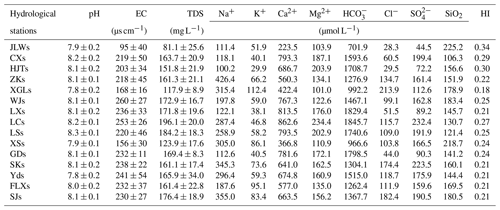Major ion compositions are shown in the Piper plot (Fig. 3). Ca2+ was the dominant cation with concentration ranging from 199 to 1107 µmol L−1, accounting for approximately 49 % to 81 %, with an average of 66 % (µEq) of the total cation composition in the river water samples. ${\mathrm{HCO}}_{\mathrm{3}}^{-}$ was the dominant anion, with concentration ranging from 640 to 2289 µmol L−1. On average, it comprised 77 % (59 %–92 %) of total anions, followed by ${\mathrm{SO}}_{\mathrm{4}}^{\mathrm{2}-}$ (16 %) and Cl (6 %). The major ionic composition indicated that the water chemistry of the Bei Jiang basin was controlled by both carbonate and silicate weathering.

The PCA was used to extract the factors controlling the chemical compositions. The varimax rotation was used to reduce the number of variables to two principal components (PCs), which together explain 76.88 % of the total variance in the data. The first PC (PC1) explained approximately 50.02 % of the total variations and was considered to represent the carbonate weathering factor because of the high loadings of EC, TDS, Ca2+, Mg2+, and ${\mathrm{HCO}}_{\mathrm{3}}^{-}$ concentrations. The second PC (PC2) explained 26.85 % of the total variance and presented high loadings for Na+ and K+ concentrations. Thus, the PC2 represented a silicate weathering factor. These two PCs were considered to be two important sources of major ions in the Bei Jiang basin.

The hydrochemical compositions of rainwater are presented in Table S1 in the Supplement. Ca2+ was the dominant cation with concentration ranging from 6.9 to 282.6 µmol L−1, accounting for approximately 65 % of the total cation composition in the rainwater samples. ${\mathrm{SO}}_{\mathrm{4}}^{\mathrm{2}-}$ was the dominant anion, with concentration ranging from 21.9 to 1462 µmol L−1, accounting for approximately 67 % of the total anion composition in the rainwater samples.

## 4.2 Seasonal and spatial variations

There were significant seasonal variations in the major ion concentrations (Fig. 4). Two basic patterns of temporal variations could be observed. The first one was related to the carbonate-weathering-derived ions such as Ca2+ and ${\mathrm{HCO}}_{\mathrm{3}}^{-}$, which showed high values in November and low values in June. The second one was for the silicate-weathering-derived ions such as Na+ and K+, which showed high values in February and low values in June. The minimums occurred in June, and all the ions showed a significant dilution effect during the high-flow periods.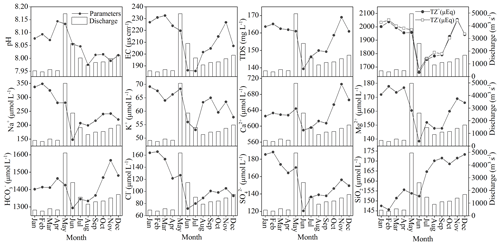Figure 4Monthly variations in environmental parameters and major ion concentrations in the Bei Jiang basin (SJs station). The columns denote the monthly discharge.

It was clear that the Ca2+ and ${\mathrm{HCO}}_{\mathrm{3}}^{-}$ concentrations had a decreasing trend from upstream to downstream (Fig. 5); this characteristic agrees with the trends observed in the Yangtze River and the Huai River, where the major elements or TDS concentrations of the main channel showed a general decreasing trend, and the tributaries display the dilution effect to the main channel. For other silicate-weathering-derived ions such as Na+, there was a slight increasing trend implying the chemical inputs from the tributaries. These trends were in accordance with the lithology in the study area. The carbonate dominates in the upper stream basin; when the river drains this area, carbonate weathering contributes to the elevation of Ca2+ and ${\mathrm{HCO}}_{\mathrm{3}}^{-}$. As the river enters into the downstream dominated by silicate, the relatively low ion concentrations due to silicate weathering contributed to diluting the Ca2+ and introducing extra Na+ to the main channel.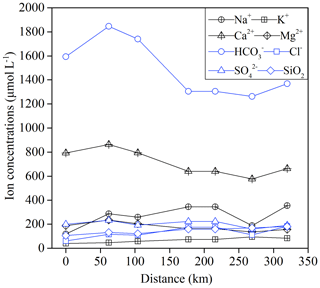Figure 5Spatial variations in major ion and SiO2 concentrations in the Bei Jiang basin (from upstream station CXs to the downstream station SJs).

5 Discussion

## 5.1 Chemical weathering rates and the controlling factors

### 5.1.1 Chemical weathering rates

Atmospheric precipitation inputs, anthropogenic inputs (here referring to the acid deposition and AMD), and chemical weathering of rocks and minerals are the major sources contributing to the hydrochemistry in the river basin. Previous studies have shown that rock weathering contributions to major element compositions of the river can be interpreted in terms of mixing among three main end-members: the weathering products of carbonates, silicates, and evaporites (Cao et al., 2016b; Négrel et al., 1993; Ollivier et al., 2010). The river water samples in the Bei Jiang basin are displayed on the plots of Na-normalized molar ratios (Fig. 6). In these plots, the contributions from carbonate weathering correspond to the trend toward the high-Ca2+ end-member close to the top right corner, while silicate weathering corresponds to the trend toward the high-Na+ end-member close to the lower left corner. It was clear that the samples with high ratio of carbonate outcrop had the highest molar ratios of ${\mathrm{Ca}}^{\mathrm{2}+}/{\mathrm{Na}}^{+}$, ${\mathrm{Mg}}^{\mathrm{2}+}/{\mathrm{Na}}^{+}$, and ${\mathrm{HCO}}_{\mathrm{3}}^{-}/{\mathrm{Na}}^{+}$, which were the samples located toward the carbonate weathering end-member. However, the samples with low ${\mathrm{Ca}}^{\mathrm{2}+}/{\mathrm{Na}}^{+}$, ${\mathrm{Mg}}^{\mathrm{2}+}/{\mathrm{Na}}^{+}$, and ${\mathrm{HCO}}_{\mathrm{3}}^{-}/{\mathrm{Na}}^{+}$ ratios showed the influence of silicate weathering. In addition, major ion compositions of the Bei Jiang were mainly contributed by the weathering of carbonates and silicates and showed little contribution of evaporite weathering.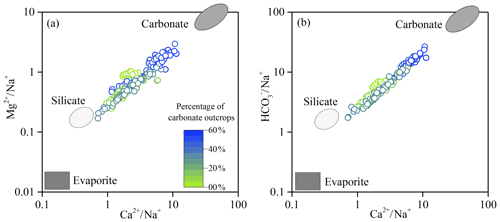Figure 6Mixing diagrams using Na-normalized molar ratios: (a) ${\mathrm{Mg}}^{\mathrm{2}+}/{\mathrm{Na}}^{+}$ vs. ${\mathrm{Ca}}^{\mathrm{2}+}/{\mathrm{Na}}^{+}$ and (b) ${\mathrm{HCO}}_{\mathrm{3}}^{-}/{\mathrm{Na}}^{+}$ vs. ${\mathrm{Ca}}^{\mathrm{2}+}/{\mathrm{Na}}^{+}$ for the Bei Jiang basin. The color ramp showed the percentage of carbonate outcrops.

Based on the chemical balance method, the calculated contributions of different sources to the total cationic loads are presented in Fig. 7. The results show that carbonate weathering was the most important mechanism controlling the local hydrochemistry and contributed approximately 50.06 % (10.96 %–79.96 %) of the total cationic loads. Silicate weathering and atmospheric precipitation inputs accounted for 25.71 % (5.55 %–70.38 %) and 17.92 % (0 %–46.95 %), respectively. Evaporite weathering had the minimum contribution with an average of 6.31 % (0 %–24.36 %) to the total cationic loads.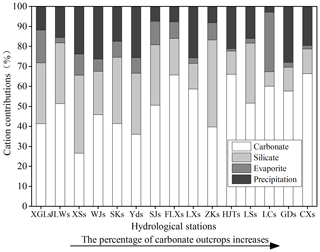Figure 7Calculated contributions (%) from the different hydrological stations to the total cationic load in the Bei Jiang basin. The cationic loads were the sum of Na+, K+, Ca2+, and Mg2+.

The result of chemical weathering rates is listed in Table 2. The carbonate weathering contributes about 70 % of the total chemical weathering, and the averages of carbonate and silicate weathering rate in the Bei Jiang basin were 61.15 and 25.31 t km−2 a−1, respectively. In addition, chemical weathering rates showed significant seasonal variations, with the highest carbonate and silicate weathering rates in May (16.75 and 5.50 t km−2 per month, respectively) and the lowest carbonate and silicate weathering rates in February (0.95 and 0.39 t km−2 per month, respectively). Gaillardet et al. (1999) reported the chemical weathering rate of major rivers all over the world and found that the hyperactive zone with a high chemical weathering rate is generally located between the latitude 0 and 30 and our study belongs to this area (Fig. 8). The factors influencing the balance between CWR and SWR will be further discussed in the following sections.

Table 2The annual discharge, catchment area, carbonate and silicate outcrop proportions, and calculated weathering rates of carbonate and silicate of 15 subcatchments in the Bei Jiang.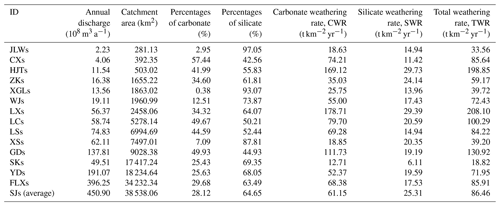### 5.1.2 Factors affecting chemical weathering

Many factors control the chemical weathering rates, including terrain, geotectonic properties, lithology, land cover, climatic conditions (temperature, precipitation, etc.), and hydrological characteristics (Ding et al., 2017; Gislason et al., 2009; Hagedorn and Cartwright, 2009). For this study, the lithology, hydrological characteristics, and geomorphology were selected as the major factors to be discussed.

### Lithology

Among all the factors controlling the chemical weathering rates, lithology is one of the most important factors because different types of rocks have different weathering abilities (Viers et al., 2014). The TWR had a significant positive correlation (p<0.01) with the ratios of the proportion of carbonate and a nonsignificant positive correlation with that of silicate outcrops (Fig. 9a, b). Furthermore, a significant correlation (p<0.01) was found between the CWR and proportion of carbonate outcrops (Fig. 9c), but the correlation between the SWR and the proportion of silicate outcrops was low and not statistically significant (p>0.05, Fig. 9d). The correlation analysis confirmed that carbonate outcrop ratios were the sensitive factor controlling the chemical weathering rates, and the rapid kinetics of carbonate dissolution played an important role in weathering rates in the Bei Jiang basin.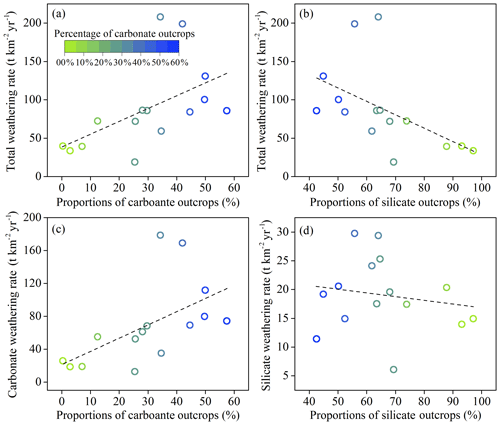Figure 9The relationships between weathering rates and the proportions of carbonate or silicate outcrops.

### Runoff

Chemical weathering is a combination of two processes, including dissolution of primary minerals and precipitation of secondary mineral growth (Eiriksdottir et al., 2011; Hartmann et al., 2014a; Liu et al., 2013). The dissolution process is related to precipitation and runoff. In general, river water chemistry is usually diluted by river runoff (Q), and this dilution effect is variable in different basins (Rao et al., 2019). The dilution effects of major elements caused by increasing water flow can be expressed by a log linear equation, the standard rating relationship (Li et al., 2014; Walling, 1986; Zhang et al., 2007):

$\begin{array}{}\text{(30)}& {C}_{i}=a{Q}^{b},\end{array}$

where Ci is the concentration of element i (mmol L−1), Q is the water discharge (m3 s−1), a is the regression constant, and b is the regression exponent. The linear fitting result is shown by Fig. 10, and the parameters b for major elements obtained from the dataset were 0.08 (Na+), 0.05 (K+), 0.08 (Ca2+), 0.02 (Mg2+), 0.06 (${\mathrm{HCO}}_{\mathrm{3}}^{-}$), 0.12 (Cl), 0.11 (${\mathrm{SO}}_{\mathrm{4}}^{\mathrm{2}-}$), and −0.005 (SiO2). In many cases, b ranges from −1 to 0 due to the chemical variables that are influenced in various ways and various extents. However, in our study area, the values of b were positive and not comparable to the observations in major Asian rivers such as the Yangtze (Chen et al., 2002), the Yellow (Chen et al., 2005), the Pearl (Zhang et al., 2007), and the Mekong (Li et al., 2014). This suggested additional and significant solute sources in the river basin that might contribute to and compensate for the effect of dilution by precipitation. The difference of slope for individual dissolved components at different stations reflected the different sources and the solubility of source materials.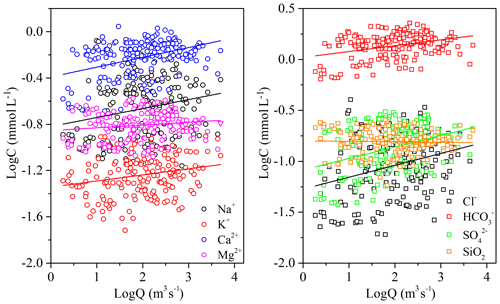Figure 10The relationship between major ion concentrations and runoff (Q) in logarithmic scales.

Due to the compensation effect of chemical weathering, significant positive linear relationship was detected between Q and TWR, CWR, and SWR. Thus, the linear regression analyses between Q and TWR, CWR, and SWR were conducted to further reveal the effect of runoff on chemical weathering rate. The slope of the liner regression equations for all 15 hydrological station watersheds in the Bei Jiang basin are summarized in Table 3. The linear relations indicated that the increase in runoff could accelerate the chemical weathering rates, but the variations in K values revealed that the degrees of influences were different due to multiple factors, such as the influence of geomorphology.

Table 3The slope of the liner regression equation between runoff (Q) and total weathering rate (TWR), carbonate weathering rate (CWR), and silicate weathering rate (SWR).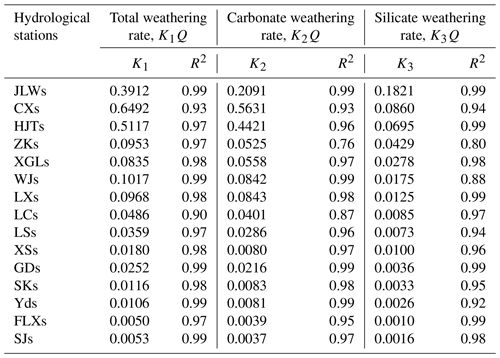### Geomorphology

The geomorphology factors including catchment area, average slope, and HI, which could influence the runoff generation process and physical and chemical weathering, were selected to give a further explanation of the variation in K values. As shown in Fig. 11a, the K values were found to have a nonlinear relationship with the areas of subcatchment and could be fitted by an exponential decay model, which showed that the K values decreased dramatically with the initial increase in area and quickly become stable after reaching the threshold. The threshold value for K1, K2, and K3 was about 5000 km2. This indicated that the compensation effect was more significant in small catchments.

The average topographic slope of each subcatchment ranged from 37 to 63. With the increasing average slope, the residence time of both surface water and groundwater decreases. Kinetics of carbonate and silicate reactions was determined by the reaction time, which could be related by the residence time of water. In our study area, the K values showed nonlinear negative correlation with average slope (Fig. 11e, f, g). When the average slope increases, the resulting small residence time (time of water–rock reactions) makes the compensation effect weak in the study area.

Hypsometric analysis showed that the HI ranged from 0.18 to 0.34. According to the empirical classification by HI (HI > 0.6, nonequilibrium or young stage; 0.35 < HI  0.6, equilibrium or mature stage; HI  0.35, monadnock or old age), the geomorphological development in the Bei Jiang was recognized as old age, which reflects that the erodible degree and erosion trend of the geomorphology were high. Furthermore, the nonlinear positive correlations between HI and K values (Fig. 11g, h, i) also addressed the fact that geomorphology development has a significant influence on chemical weathering and related CO2 consumption processes.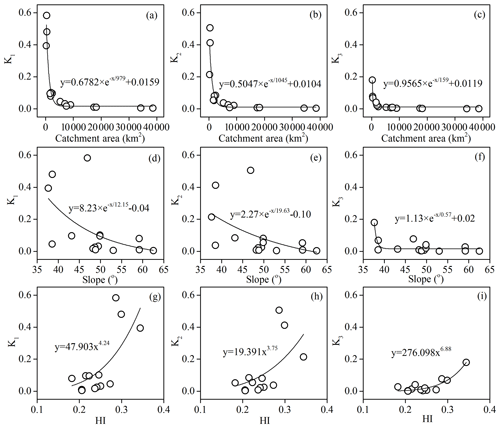Figure 11The relationships between K values and catchment area (a, b, c), average slope (d, e, f), and HI (g, h, i) for the Bei Jiang.

## 5.2 Temporary and net sink of atmospheric CO2

### 5.2.1 Sulfate origin and DIC apportionment

The successful application of DIC apportionment calculation mentioned in Sect. 3.2.2 is dependent on the origins of sulfate (${\mathrm{SO}}_{\mathrm{4}}^{\mathrm{2}-}$). Three origins of ${\mathrm{SO}}_{\mathrm{4}}^{\mathrm{2}-}$ should be discriminated, including atmospheric acid deposition (Larssen and Carmichael, 2000), acid mining discharge (AMD) (Li et al., 2018, 2019), and chemical weathering of evaporite such as the dissolution of gypsum (Appelo and Postma, 2005). Acid rain events occurred frequently in south and east China after 1980 (Larssen et al., 2006). The pH isolines based on data from 86 monitoring stations (Larssen et al., 2006) showed that in the Bei Jiang the rain pH was lower than 4.5, and our monitoring dataset also proved this result. Sulfur wet deposition estimated based on the observed bulk wet sulfur deposition data and the RAINS-Asia model (Larssen and Carmichael, 2000) ranged from 2000 to 5000 eq ha−1 a−1, which showed that the acid sulfur deposition was one of the most important sources of river sulfate. In addition, considering the abundant ore resources in the Bei Jiang, the second possible source of ${\mathrm{SO}}_{\mathrm{4}}^{\mathrm{2}-}$ is sulfide oxidation due to mining. In our previous study, the ${\mathrm{SO}}_{\mathrm{4}}^{\mathrm{2}-}$ with AMD origin mainly came from the tributary Wen Jiang (Wen et al., 2018). These two sources could offer a sufficient amount of the chemical weathering agent H2SO4 and be actively involved in the chemical weathering due to the following reaction mechanism (take carbonate for example) (Taylor et al., 1984; van Everdingen and Krouse, 1985).

$\begin{array}{}\text{(31)}& {\mathrm{FeS}}_{\mathrm{2}}+\frac{\mathrm{7}}{\mathrm{2}}{\mathrm{O}}_{\mathrm{2}}+{\mathrm{H}}_{\mathrm{2}}\mathrm{O}={\mathrm{Fe}}^{\mathrm{2}+}+\mathrm{2}{\mathrm{SO}}_{\mathrm{4}}^{\mathrm{2}-}+\mathrm{2}{\mathrm{H}}^{+}\text{(32)}& \mathrm{2}{\mathrm{CaCO}}_{\mathrm{3}}+{\mathrm{H}}_{\mathrm{2}}{\mathrm{SO}}_{\mathrm{4}}=\mathrm{2}{\mathrm{Ca}}^{\mathrm{2}+}+\mathrm{2}{\mathrm{HCO}}_{\mathrm{3}}^{-}+{\mathrm{SO}}_{\mathrm{4}}^{\mathrm{2}-}\end{array}$

The third source came from dissolution of gypsum and could not offer active H2SO4 to induce carbonate and silicate dissolution. Two pieces of evidence were summarized to indicate the absence of gypsum in the study area. (1) Lithology in the river basin is composed of limestone, sandstone, gneiss, and glutenite. HI showed that geomorphology development has entered into the old age, and the evaporite such as halite and gypsum has been consumed by dissolution. (2) The stoichiometric relationship between Ca2+ and ${\mathrm{SO}}_{\mathrm{4}}^{\mathrm{2}-}$ (Fig. 2) showed that all of the samples in the study area were located below the 1 : 1 gypsum dissolution line, and they are also below the 1 : 2 carbonate weathering line induced by sulfuric acid (SCW). These two points combined gave the evidence to prove the absence of contribution of gypsum dissolution to river ${\mathrm{SO}}_{\mathrm{4}}^{\mathrm{2}-}$. Thus, the DIC apportionment could be calculated according to Eqs. (23) to (25), and the results of the three main processes (CCW, CSW, and SCW) contributing to the DIC origin in the Bei Jiang water are shown in Table 4. It was found that CCW was the dominant origin of DIC (35 %–87 %) and that SCW (3 %–15 %) and CSW (7 %–59 %) were non-negligible weathering processes.

### 5.2.2 Temporary and net CO2 sink

According to the classical view of the global carbon cycling (Berner and Kothavala, 2001), the CO2 sink induced by chemical weathering varies for different timescales. At a short-term timescale, carbonic-acid-based carbonate and silicate weathering (CCW and CSW) and transport of the ${\mathrm{HCO}}_{\mathrm{3}}^{-}$ to oceans through rivers is an important temporary carbon sink (Khadka et al., 2014) and can be calculated by the sum of CCRCCW and CCRCSW. Thus, it was significant to estimate the CCR of CCW and CSW (Liu and Dreybrodt, 2015; Liu et al., 2011). However, at the geological timescale (>106 years), over the timescale typical of the residence time of ${\mathrm{HCO}}_{\mathrm{3}}^{-}$ in the ocean (105 years), the CCW is not a mechanism that can participate in the net sink of CO2 in the atmosphere because all of the atmospheric CO2 fixed through CCW is returned to the atmosphere during carbonate precipitation in the ocean. Meanwhile, in the case of CSW, followed by carbonate deposition, 1 of the 2 mol of CO2 involved is transferred from the atmosphere to the lithosphere in the form of carbonate rocks, while the other 1 mol returns to the atmosphere. The CSW is recognized as the net sink of atmosphere CO2. In addition, when sulfuric acid is involved as a proton donor in carbonate weathering, half of the carbon is dissolved to the atmosphere during carbonate precipitation. Thus, SCW leads to a net release of CO2 in the ocean–atmosphere system. Thus the net CO2 sink (expressed by CCRNet in this study) is controlled by the DIC apportionment according to Eq. (28).

Table 4Calculated CO2 consumption rate and net sink of 15 nested subcatchments in the Bei Jiang basin.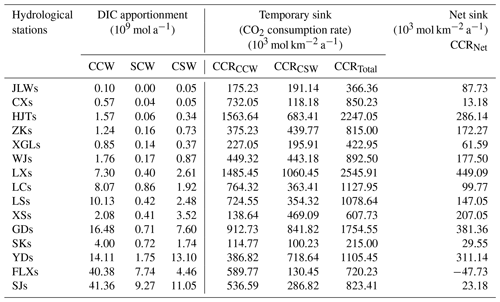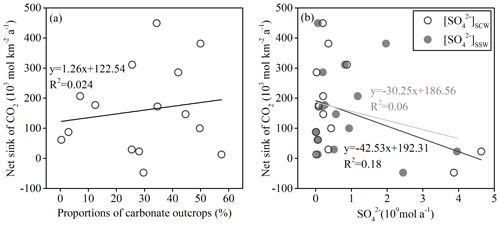Figure 12Correlations between CO2 net sinks and proportions of carbonate (a) and correlations between CO2 net sinks and [${\mathrm{SO}}_{\mathrm{4}}^{\mathrm{2}-}$]SCW or [${\mathrm{SO}}_{\mathrm{4}}^{\mathrm{2}-}$]SSW (b).

The results of CCRTotal, CCRCCW, CCRCSW, and CCRNet were summarized in Table 4. The CCRTotal was 823.41×103 mol km−2 a−1. Comparing with other Chinese rivers, such as the Songhua River (189×103 mol km−2 a−1) (Cao et al., 2015) and other rivers calculated by Gaillardet et al. (1999) including the Heilong River (53×103 mol km−2 a−1), the Yangtze River (609×103 mol km−2 a−1), the Huang He (360×103 mol km−2 a−1), the Xi Jiang (960×103 mol km−2 a−1), the Jinsha Jiang (420×103 mol km−2 a−1), the Langcang Jiang (980×103 mol km−2 a−1), the Nu Jiang (1240×103 mol km−2 a−1), the Yalong Jiang (870×103 mol km−2 a−1), the Dadu He (1280×103 mol km−2 a−1), and Min Jiang (660×103 mol km−2 a−1), our study area showed relatively high CCR due to a high chemical weathering rate. In addition, the CCRCCW and CCRCSW were 536.59×103 (65 %) and 286.82×103 (35 %) mol km−2 a−1, respectively. Compared with the temporary sink, the net sink of CO2 for the Bei Jiang was approximately 23.18×103 mol km−2 a−1 of CO2 sinking in the global carbon cycling. It was about 3 % of the temporary CO2 sink. In addition, the CO2 net sink of each subbasin was also different and showed large spatial variations due to heterogeneity of geology and human activities. The geology showed weak correlation with the CO2 net sink (Fig. 12a), while the [${\mathrm{SO}}_{\mathrm{4}}^{\mathrm{2}-}$]SCW and [${\mathrm{SO}}_{\mathrm{4}}^{\mathrm{2}-}$]SSW have weak negative correlation with the CO2 net sink (Fig. 12b). This proved that human activities (sulfur acid deposition and AMD) decreased the CO2 net sink and even made chemical weathering a CO2 source to the atmosphere.

6 Conclusions

This study revealed the temporary and net sinks of atmospheric CO2 due to chemical weathering in a subtropical hyperactive catchment with mixing carbonate and silicate lithology under the stress of chemical weathering induced by anthropogenic sulfuric acid agent. During the sampling period, the pH values ranged from 7.5 to 8.5 and TDS varied from 73.8 to 230.2 mg L−1. Ca2+ and ${\mathrm{HCO}}_{\mathrm{3}}^{-}$ were the dominant cation and anion. Water chemical patterns and PCA showed that carbonate and silicate weathering were the most important processes controlling the local hydrochemistry. On average, carbonate and silicate weathering contributed approximately 50.06 % and 25.71 % of the total cationic loads, respectively.

The averages of carbonate and silicate weathering rate in the Bei Jiang basin were 61.15 and 25.31 t km−2 a−1, respectively. The high rate was comparable to other rivers located in the hyperactive zone between the latitude 0 and 30. The lithology, runoff, and geomorphology had significant influences on the chemical weathering rate. (1) Due to the difference between kinetics of carbonate and silicate dissolution processes, the proportion of carbonate outcrops had significant positive correlation with the chemical weathering rate and confirmed that carbonate outcrop ratios were the sensitive factor controlling the chemical weathering rates, and the rapid kinetics of carbonate dissolution played an important role in weathering rates. (2) Runoff mainly controlled the season variations, and the dilution effect was weak in the study area. Due to the compensation effect of chemical weathering, a significant positive linear relationship was detected between Q and TWR, CWR, and SWR. (3) The geomorphology factors such as slope and HI had a nonlinear correlation with chemical weathering rate and showed a significant scale effect, which revealed the complexity in chemical weathering processes.

DIC apportionment showed that CCW was the dominant origin of DIC (35 %–87 %) and that SCW (3 %–15 %) and CSW (7 %–59 %) were non-negligible weathering processes. The CCRTotal was 823.41×103 mol km−2 a−1, relatively high CCR due to a high chemical weathering rate. In addition, the CCRCCW and CCRCSW were 536.59×103 (65 %) and 286.82×103 (35 %) mol km−2 a−1, respectively. Compared with the temporary sink, the net sink of CO2 for the Bei Jiang was approximately 23.18×103 mol km−2 a−1 of CO2 sinking in global carbon cycling. It was about 2.82 % of the temporary CO2 sink. Human activities such as sulfur acid deposition and AMD have significantly altered the CO2 sinks.

Data availability
Data availability.

The data in this study have been presented in the tables of the article and can also be requested from the corresponding author.

Supplement
Supplement.

Author contributions
Author contributions.

YC and CT designed the study, carried out the fieldwork, analyzed the results, and drafted the manuscript. YX and SG participated in the field sampling and laboratory analysis. YP reviewed and edited the original draft of the manuscript. All authors read and approved the final manuscript.

Competing interests
Competing interests.

The authors declare that they have no conflict of interest.

Acknowledgements
Acknowledgements.

This research work was financially supported by the General Program of the National Natural Science Foundation of China (no. 41877470) and the Natural Science Foundation of Guangdong Province, China (nos. 2017A030313231, 2017A030313229).

Financial support
Financial support.

This research has been supported by the General Program of the National Natural Science Foundation of China (grant no. 41877470) and the Natural Science Foundation of Guangdong Province, China (grant nos. 2017A030313231 and 2017A030313229).

Review statement
Review statement.

This paper was edited by Nobuhito Ohte and reviewed by two anonymous referees.

References

Appelo, C. A. J. and Postma, D.: Geochemistry, groundwater and pollution, CRC press, Boca Raton, United States, 2005.

Berner, R. A. and Kothavala, Z.: GEOCARB III: a revised model of atmospheric CO2 over Phanerozoic time, Am. J. Sci., 301, 182–204, 2001.

Cao, Y., Tang, C., Song, X., and Liu, C.: Major ion chemistry, chemical weathering and CO2 consumption in the Songhua River basin, Northeast China, Environ. Earth Sci., 73, 7505–7516, 2015.

Cao, Y., Tang, C., Song, X., Liu, C., and Zhang, Y.: Identifying the hydrochemical characteristics of rivers and groundwater by multivariate statistical analysis in the Sanjiang Plain, China, Appl. Water Sci., 6, 169–178, 2016a.

Cao, Y., Tang, C., Cao, G., and Wang, X.: Hydrochemical zoning: natural and anthropogenic origins of the major elements in the surface water of Taizi River Basin, Northeast China, Environ. Earth Sci., 75, 1–14, 2016b.

Chen, J., Wang, F., Xia, X., and Zhang, L.: Major element chemistry of the Changjiang (Yangtze River), Chem. Geol., 187, 231–255, 2002.

Chen, J., Wang, F., Meybeck, M., He, D., Xia, X., and Zhang, L.: Spatial and temporal analysis of water chemistry records (1958–2000) in the Huanghe (Yellow River) basin, Global Biogeochem. Cy., 19, GB3016, https://doi.org/10.1029/2004GB002325, 2005.

Ding, H., Liu, C.-Q., Zhao, Z.-Q., Li, S.-L., Lang, Y.-C., Li, X.-D., Hu, J., and Liu, B.-J.: Geochemistry of the dissolved loads of the Liao River basin in northeast China under anthropogenic pressure: Chemical weathering and controlling factors, J. Asian Earth Sci., 138, 657–671, https://doi.org/10.1016/j.jseaes.2016.07.026, 2017.

Donnini, M., Frondini, F., Probst, J.-L., Probst, A., Cardellini, C., Marchesini, I., and Guzzetti, F.: Chemical weathering and consumption of atmospheric carbon dioxide in the Alpine region, Global Planet. Change, 136, 65–81, https://doi.org/10.1016/j.gloplacha.2015.10.017, 2016.

Dosseto, A., Bourdon, B., Gaillardet, J., Allègre, C. J., and Filizola, N.: Time scale and conditions of weathering under tropical climate: Study of the Amazon basin with U-series, Geochim. Cosmochim. Ac., 70, 71–89, https://doi.org/10.1016/j.gca.2005.06.033, 2006.

Edmond, J. M., Palmer, M. R., Measures, C. I., Brown, E. T., and Huh, Y.: Fluvial geochemistry of the eastern slope of the northeastern Andes and its foredeep in the drainage of the Orinoco in Colombia and Venezuela, Geochim. Cosmochim. Ac., 60, 2949–2974, https://doi.org/10.1016/0016-7037(96)00142-1, 1996.

Eiriksdottir, E. S., Gislason, S. R., and Oelkers, E. H.: Does runoff or temperature control chemical weathering rates?, Appl. Geochem., 26, S346–S349, https://doi.org/10.1016/j.apgeochem.2011.03.056, 2011.

Fernandes, A. M., Conceição, F. T. d., Spatti Junior, E. P., Sardinha, D. d. S., and Mortatti, J.: Chemical weathering rates and atmospheric/soil CO2 consumption of igneous and metamorphic rocks under tropical climate in southeastern Brazil, Chem. Geol., 443, 54–66, https://doi.org/10.1016/j.chemgeo.2016.09.008, 2016.

Gaillardet, J., Dupré, B., Louvat, P., and Allègre, C. J.: Global silicate weathering and CO2 consumption rates deduced from the chemistry of large rivers, Chem. Geol., 159, 3–30, https://doi.org/10.1016/S0009-2541(99)00031-5, 1999.

Galy, A. and France-Lanord, C.: Weathering processes in the Ganges–Brahmaputra basin and the riverine alkalinity budget, Chem. Geol., 159, 31–60, https://doi.org/10.1016/S0009-2541(99)00033-9, 1999.

Gao, Q., Tao, Z., Huang, X., Nan, L., Yu, K., and Wang, Z.: Chemical weathering and CO2 consumption in the Xijiang River basin, South China, Geomorphology, 106, 324–332, https://doi.org/10.1016/j.geomorph.2008.11.010, 2009.

Garrels, R. M.: The carbonate-silicate geochemical cycle and its effect on atmospheric carbon dioxide over the past 100 million years, Am. J. Sci., 283, 641–683, 1983.

Gibbs, R. J.: Water chemistry of the Amazon River, Geochim. Cosmochim. Ac., 36, 1061–1066, https://doi.org/10.1016/0016-7037(72)90021-X, 1972.

Gislason, S. R., Oelkers, E. H., Eiriksdottir, E. S., Kardjilov, M. I., Gisladottir, G., Sigfusson, B., Snorrason, A., Elefsen, S., Hardardottir, J., Torssander, P., and Oskarsson, N.: Direct evidence of the feedback between climate and weathering, Earth Planet. Sc. Lett., 277, 213–222, https://doi.org/10.1016/j.epsl.2008.10.018, 2009.

Guo, J., Wang, F., Vogt, R. D., Zhang, Y., and Liu, C. Q.: Anthropogenically enhanced chemical weathering and carbon evasion in the Yangtze Basin, Sci. Rep.-UK, 5, 11941, https://doi.org/10.1038/srep11941, 2015.

Hagedorn, B. and Cartwright, I.: Climatic and lithologic controls on the temporal and spatial variability of CO2 consumption via chemical weathering: An example from the Australian Victorian Alps, Chem. Geol., 260, 234–253, https://doi.org/10.1016/j.chemgeo.2008.12.019, 2009.

Hartmann, J., Jansen, N., Dürr, H. H., Kempe, S., and Köhler, P.: Global CO2-consumption by chemical weathering: What is the contribution of highly active weathering regions?, Global Planet. Change, 69, 185–194, https://doi.org/10.1016/j.gloplacha.2009.07.007, 2009.

Hartmann, J., Moosdorf, N., Lauerwald, R., Hinderer, M., and West, A. J.: Global chemical weathering and associated P-release – The role of lithology, temperature and soil properties, Chem. Geol., 363, 145–163, https://doi.org/10.1016/j.chemgeo.2013.10.025, 2014a.

Hartmann, J., West, J., Renforth, P., Köhler, P., Rocha, C. D. L., Wolf-Gladrow, D., Dürr, H., and Scheffran, J.: Enhanced chemical weathering as a sink for carbon dioxide, a nutrient source and a strategy to mitigate ocean acidification, Rev. Geophys., 51, 113–149, https://doi.org/10.1002/org.20004, 2014b.

He, J. Y., Zhang, D., and Zhao, Z. Q.: Spatial and temporal variations in hydrochemical composition of river water in Yellow River Basin, China, Chinese J. Ecol., 36, 1390–1401, 2017.

Hercod, D. J., Brady, P. V., and Gregory, R. T.: Catchment-scale coupling between pyrite oxidation and calcite weathering, Chem. Geol., 151, 259–276, https://doi.org/10.1016/S0009-2541(98)00084-9, 1998.

Huh, Y. and Edmond, J. M.: The fluvial geochemistry of the rivers of Eastern Siberia: III. Tributaries of the Lena and Anabar draining the basement terrain of the Siberian Craton and the Trans-Baikal Highlands, Geochim. Cosmochim. Ac., 63, 967–987, https://doi.org/10.1016/S0016-7037(99)00045-9, 1999.

Jiang, H., Liu, W., Xu, Z., Zhou, X., Zheng, Z., Zhao, T., Zhou, L., Zhang, X., Xu, Y., and Liu, T.: Chemical weathering of small catchments on the Southeastern Tibetan Plateau I: Water sources, solute sources and weathering rates, Chem. Geol., 500, 159–174, https://doi.org/10.1016/j.chemgeo.2018.09.030, 2018.

Kempe, S. and Degens, E. T.: An early soda ocean?, Chem. Geol., 53, 95–108, https://doi.org/10.1016/0009-2541(85)90023-3, 1985.

Khadka, M. B., Martin, J. B., and Jin, J.: Transport of dissolved carbon and CO2 degassing from a river system in a mixed silicate and carbonate catchment, J. Hydrol., 513, 391–402, https://doi.org/10.1016/j.jhydrol.2014.03.070, 2014.

Larssen, T. and Carmichael, G. R.: Acid rain and acidification in China: the importance of base cation deposition, Environ. Pollut., 110, 89–102, https://doi.org/10.1016/S0269-7491(99)00279-1, 2000.

Larssen, T., Lydersen, E., Tang, D., He, Y., Gao, J., Liu, H., Duan, L., Seip, H. M., Vogt, R. D., Mulder, J., Shao, M., Wang, Y., Shang, H., Zhang, X., Solberg, S., Aas, W., Okland, T., Eilertsen, O., Angell, V., Li, Q., Zhao, D., Xiang, R., Xiao, J., and Luo, J.: Acid Rain in China, Environ. Sci. Technol., 40, 418–425, https://doi.org/10.1021/es0626133, 2006.

Lenton, T. M. and Britton, C.: Enhanced carbonate and silicate weathering accelerates recovery from fossil fuel CO2 perturbations, Global Biogeochem. Cy., 20, GB3009, https://doi.org/10.1029/2005gb002678, 2006.

Li, R., Tang, C., Cao, Y., Jiang, T., and Chen, J.: The distribution and partitioning of trace metals (Pb, Cd, Cu, and Zn) and metalloid (As) in the Beijiang River, Environ. Monit. Assess., 190, 399, https://doi.org/10.1007/s10661-018-6789-x, 2018.

Li, R., Tang, C., Li, X., Jiang, T., Shi, Y., and Cao, Y.: Reconstructing the historical pollution levels and ecological risks over the past sixty years in sediments of the Beijiang River, South China, Sci. Total Environ., 649, 448–460, https://doi.org/10.1016/j.scitotenv.2018.08.283, 2019.

Li, S., Lu, X. X., He, M., Zhou, Y., Bei, R., Li, L., and Ziegler, A. D.: Major element chemistry in the upper Yangtze River: A case study of the Longchuanjiang River, Geomorphology, 129, 29–42, https://doi.org/10.1016/j.geomorph.2011.01.010, 2011.

Li, S., Lu, X. X., and Bush, R. T.: Chemical weathering and CO2 consumption in the Lower Mekong River, Sci. Total Environ., 472, 162–177, https://doi.org/10.1016/j.scitotenv.2013.11.027, 2014.

Li, S. L., Calmels, D., Han, G., Gaillardet, J., and Liu, C. Q.: Sulfuric acid as an agent of carbonate weathering constrained by δ13CDIC: Examples from Southwest China, Earth Planet. Sc. Lett., 270, 189–199, https://doi.org/10.1016/j.epsl.2008.02.039, 2008.

Liu, B., Liu, C.-Q., Zhang, G., Zhao, Z.-Q., Li, S.-L., Hu, J., Ding, H., Lang, Y.-C., and Li, X.-D.: Chemical weathering under mid- to cool temperate and monsoon-controlled climate: A study on water geochemistry of the Songhuajiang River system, northeast China, Appl. Geochem., 31, 265–278, https://doi.org/10.1016/j.apgeochem.2013.01.015, 2013.

Liu, Z. and Dreybrodt, W.: Significance of the carbon sink produced by H2O–carbonate–CO2–aquatic phototroph interaction on land, Sci. Bull., 60, 182–191, 2015.

Liu, Z., Dreybrodt, W., and Liu, H.: Atmospheric CO2 sink: Silicate weathering or carbonate weathering?, Appl. Geochem., 26, S292–S294, https://doi.org/10.1016/j.apgeochem.2011.03.085, 2011.

Ludwig, W., Amiotte-Suchet, P., Munhoven, G., and Probst, J.-L.: Atmospheric CO2 consumption by continental erosion: present-day controls and implications for the last glacial maximum, Global Planet. Change, 16–17, 107–120, https://doi.org/10.1016/S0921-8181(98)00016-2, 1998.

Meybeck, M., Dürr, H. H., and Vörösmarty, C. J.: Global coastal segmentation and its river catchment contributors: A new look at land-ocean linkage, Global Biogeochem. Cy., 20, GB1S90, https://doi.org/10.1029/2005gb002540, 2006.

Mora, A., Baquero, J. C., Alfonso, J. A., Pisapia, D., and Balza, L.: The Apure River: geochemistry of major and selected trace elements in an Orinoco River tributary coming from the Andes, Venezuela, Hydrol. Process., 24, 3798–3810, https://doi.org/10.1002/hyp.7801, 2010.

Mortatti, J. and Probst, J.-L.: Silicate rock weathering and atmospheric/soil CO2 uptake in the Amazon basin estimated from river water geochemistry: seasonal and spatial variations, Chem. Geol., 197, 177–196, https://doi.org/10.1016/S0009-2541(02)00349-2, 2003.

Négrel, P., Allègre, C. J., Dupré, B., and Lewin, E.: Erosion sources determined by inversion of major and trace element ratios and strontium isotopic ratios in river water: The Congo Basin case, Earth and Planet. Sci. Lett., 120, 59–76, https://doi.org/10.1016/0012-821X(93)90023-3, 1993.

Ollivier, P., Hamelin, B., and Radakovitch, O.: Seasonal variations of physical and chemical erosion: A three-year survey of the Rhone River (France), Geochim. Cosmochim. Ac., 74, 907–927, https://doi.org/10.1016/j.gca.2009.10.037, 2010.

Pike, R. J. and Wilson, S. E.: Elevation-Relief Ratio, Hypsometric Integral, and Geomorphic Area-Altitude Analysis, GSA Bulletin, 82, 1079–1084, https://doi.org/10.1130/0016-7606(1971)82[1079:erhiag]2.0.co;2, 1971.

Ran, X., Yu, Z., Yao, Q., Chen, H., and Mi, T.: Major ion geochemistry and nutrient behaviour in the mixing zone of the Changjiang (Yangtze) River and its tributaries in the Three Gorges Reservoir, Hydrol. Process., 24, 2481–2495, 2010.

Rao, W., Zheng, F., Tan, H., Yong, B., Jin, K., Wang, S., Zhang, W., Chen, T., and Wang, Y.: Major ion chemistry of a representative river in South-central China: Runoff effects and controlling mechanisms, J. Hazard. Mater., 378, 120755, https://doi.org/10.1016/j.jhazmat.2019.120755, 2019.

Ryu, J. S., Lee, K. S., Chang, H.-W., and Shin, H. S.: Chemical weathering of carbonates and silicates in the Han River basin, South Korea, Chem. Geol., 247, 66–80, https://doi.org/10.1016/j.chemgeo.2007.09.011, 2008.

Singh, O., Sarangi, A., and Sharma, M. C.: Hypsometric Integral Estimation Methods and its Relevance on Erosion Status of North-Western Lesser Himalayan Watersheds, Water Resour. Manage., 22, 1545–1560, https://doi.org/10.1007/s11269-008-9242-z, 2008.

Spence, J. and Telmer, K.: The role of sulfur in chemical weathering and atmospheric CO2 fluxes: Evidence from major ions, δ13CDIC, and δ34S${}_{{\mathrm{SO}}_{\mathrm{4}}}$ in rivers of the Canadian Cordillera, Geochim. Cosmochim. Ac., 69, 5441–5458, https://doi.org/10.1016/j.gca.2005.07.011, 2005.

Stallard, R. F. and Edmond, J. M.: Geochemistry of the Amazon: 1. Precipitation chemistry and the marine contribution to the dissolved load at the time of peak discharge, J. Geophys. Res.-Oceans, 86, 9844–9858, https://doi.org/10.1029/JC086iC10p09844, 1981.

Stallard, R. F. and Edmond, J. M.: Geochemistry of the Amazon: 2. The influence of geology and weathering environment on the dissolved load, J. Geophys. Res.-Oceans, 88, 9671–9688, https://doi.org/10.1029/JC088iC14p09671, 1983.

Stallard, R. F. and Edmond, J. M.: Geochemistry of the Amazon: 3. Weathering chemistry and limits to dissolved inputs, J. Geophys. Res.-Oceans, 92, 8293–8302, https://doi.org/10.1029/JC092iC08p08293, 1987.

Strahler, A. N.: HYPSOMETRIC (AREA-ALTITUDE) ANALYSIS OF EROSIONAL TOPOGRAPHY, GSA Bulletin, 63, 1117–1142, https://doi.org/10.1130/0016-7606(1952)63[1117:haaoet]2.0.co;2, 1952.

Sun, X., Mörth, C.-M., Humborg, C., and Gustafsson, B.: Temporal and spatial variations of rock weathering and CO2 consumption in the Baltic Sea catchment, Chem. Geol., 466, 57–69, https://doi.org/10.1016/j.chemgeo.2017.04.028, 2017.

Taylor, B. E., Wheeler, M. C., and Nordstrom, D. K.: Stable isotope geochemistry of acid mine drainage: Experimental oxidation of pyrite, Geochim. Cosmochim. Ac., 48, 2669–2678, https://doi.org/10.1016/0016-7037(84)90315-6, 1984.

Van Everdingen, R. O. and Krouse, H. R.: Isotope composition of sulphates generated by bacterial and abiological oxidation, Nature, 315, 395–396, https://doi.org/10.1038/315395a0, 1985.

Viers, J., Oliva, P., Dandurand, J. L., Dupré, B., and Gaillardet, J.: Chemical weathering rates, CO2 consumption, and control parameters deduced from the chemical composition of rivers, Treatise on Geochemistry, 2nd Edn., Vol. 5, 1–25, https://doi.org/10.1016/B978-0-08-095975-7.00506-4, 2014.

Walling, D. E.: Solute in river systems, Solute Processes, edited by: Trudgill, S. T., 251–327, Wiley, Cichester, 1986.

Wen, J., Tang, C., Cao, Y., Li, X., and Chen, Q.: Hydrochemical evolution of groundwater in a riparian zone affected by acid mine drainage (AMD), South China: the role of river–groundwater interactions and groundwater residence time, Environ. Earth Sci., 77, 794, https://doi.org/10.1007/s12665-018-7977-2, 2018.

Wu, W., Xu, S., Yang, J., and Yin, H.: Silicate weathering and CO2 consumption deduced from the seven Chinese rivers originating in the Qinghai-Tibet Plateau, Chem. Geol., 249, 307–320, 2008.

Xie, C., Gao, Q., Tao, Z., Liu, L., and Li, S.: Chemical weathering and CO2 consumption in the Dongjiang River Basin, Acta Scientiae Circumstantiae, 33, 2123–2133, 2013.

Xiong, L., Tang, G., Yuan, B., Lu, Z., Li, F., and Zhang, L.: Geomorphological inheritance for loess landform evolution in a severe soil erosion region of Loess Plateau of China based on digital elevation models, Sci. China Earth Sci., 57, 1944–1952, https://doi.org/10.1007/s11430-014-4833-4, 2014.

Xu, Z. and Liu, C.-Q.: Water geochemistry of the Xijiang basin rivers, South China: Chemical weathering and CO2 consumption, Appl. Geochem., 25, 1603–1614, 2010.

Zeng, C., Liu, Z., Zhao, M., and Yang, R.: Hydrologically-driven variations in the karst-related carbon sink fluxes: Insights from high-resolution monitoring of three karst catchments in Southwest China, J. Hydrol., 533, 74–90, https://doi.org/10.1016/j.jhydrol.2015.11.049, 2016.

Zhang, J., Huang, W., Letolle, R., and Jusserand, C.: Major element chemistry of the Huanghe (Yellow River), China-weathering processes and chemical fluxes, J. Hydrol., 168, 173–203, 1995.

Zhang, L., Song, X., Xia, J., Yuan, R., Zhang, Y., Liu, X., and Han, D.: Major element chemistry of the Huai River basin, China, Appl. Geochem., 26, 293–300, 2011.

Zhang, S. R., Lu, X. X., Higgitt, D. L., Chen, C. T. A., Sun, H. G., and Han, J. T.: Water chemistry of the Zhujiang (Pearl River): natural processes and anthropogenic influences, J. Geophys. Res.-Atmos., 112, 137–161, 2007.